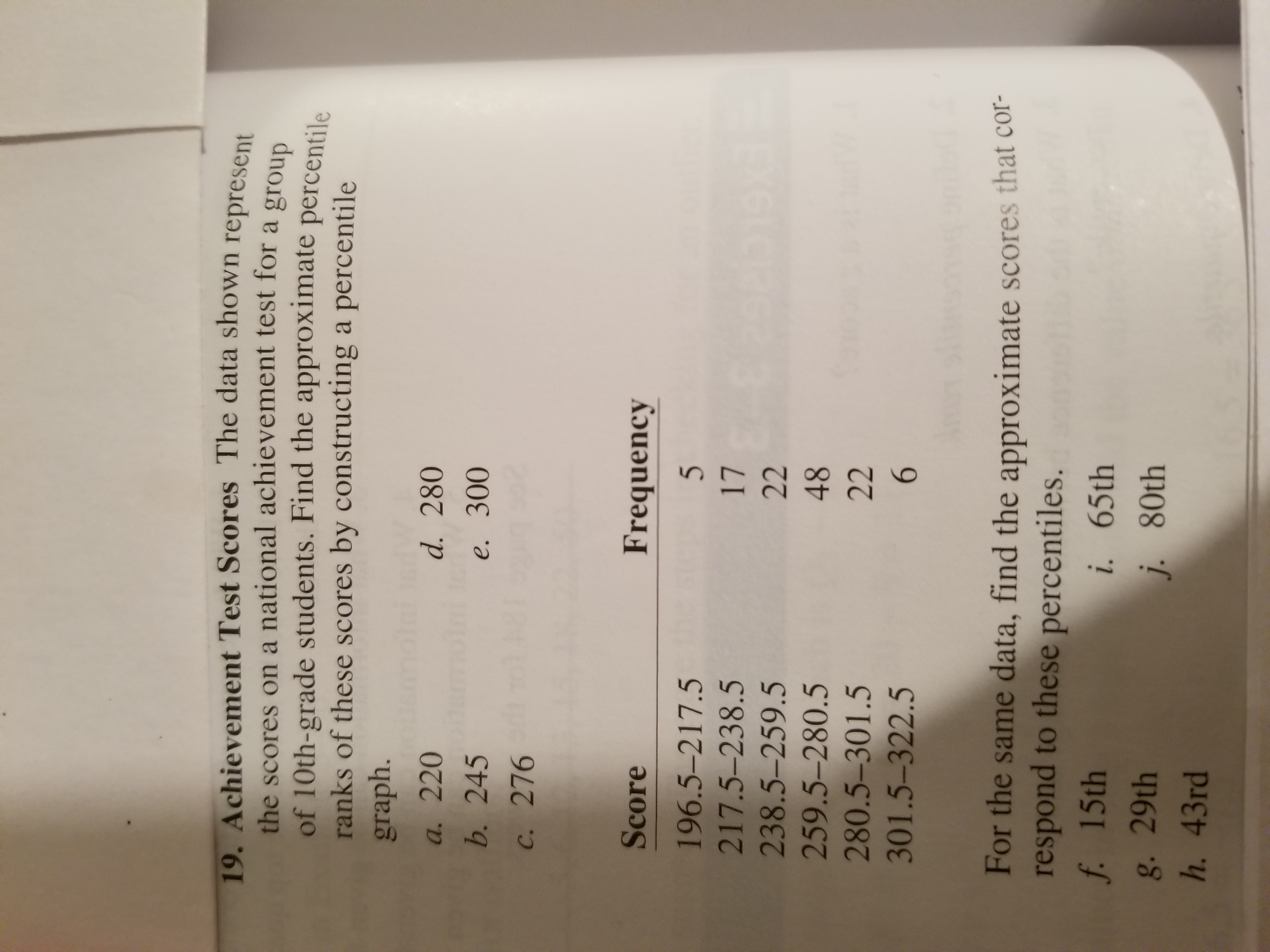# 19. Achievement Test Scores The data shown representhe scores on a national achievement test for a groupof 10th-grade students. Find the approximate percentileranks of these scores by constructing a percentilegraph.a. 220b. 245c. 276d. 280e. 300Score196.5-217.5217.5-238.5238.5-259.5259.5-280.5280.5-301.5301.5-322.5Frequency1748For the same data, find the approximate scores that cor-respond to these percentiles.f 15thg. 29thh. 43rdi. 65thj. 80th

Questionhelp_outlineImage Transcriptionclose19. Achievement Test Scores The data shown represen the scores on a national achievement test for a group of 10th-grade students. Find the approximate percentile ranks of these scores by constructing a percentile graph. a. 220 b. 245 c. 276 d. 280 e. 300 Score 196.5-217.5 217.5-238.5 238.5-259.5 259.5-280.5 280.5-301.5 301.5-322.5 Frequency 17 48 For the same data, find the approximate scores that cor- respond to these percentiles. f 15th g. 29th h. 43rd i. 65th j. 80th fullscreen
check_circleExpert Solution
Step 1

As there are lot of subparts in the question, I can only help you with the first three subparts (a,b,c) of the question. Using the percetiles calculated you can solve the next two subparts d,e.  For help in subparts f to h kindly ask a new question. Thank you

Given frequency distribution of scores on a national achievement test for a group of tenth grade students. The frequency distribution is shown below and we need to find the percentile ranks for this data.

Step 2

Here let us calculate the cumulative frequency of the data distribution. In cumulative frequency we add the frequencies of all the prior class intervals as well. For example cumulative frequency of class 196.5-217.5 is 5 and class 217.5 - 238.5 cumulative frequency is 22 and so on. After calculating the cumulative frequencies we get the following.

Step 3

Here we calculate the percentile rank of every class interval by dividing the cumulative frequency by the total frequency and multiplying by 100. Percentile rank of a score is nothing but t...

### Want to see the full answer?

See Solution

#### Want to see this answer and more?

Solutions are written by subject experts who are available 24/7. Questions are typically answered within 1 hour*

See Solution
*Response times may vary by subject and question
Tagged in

### Statistics